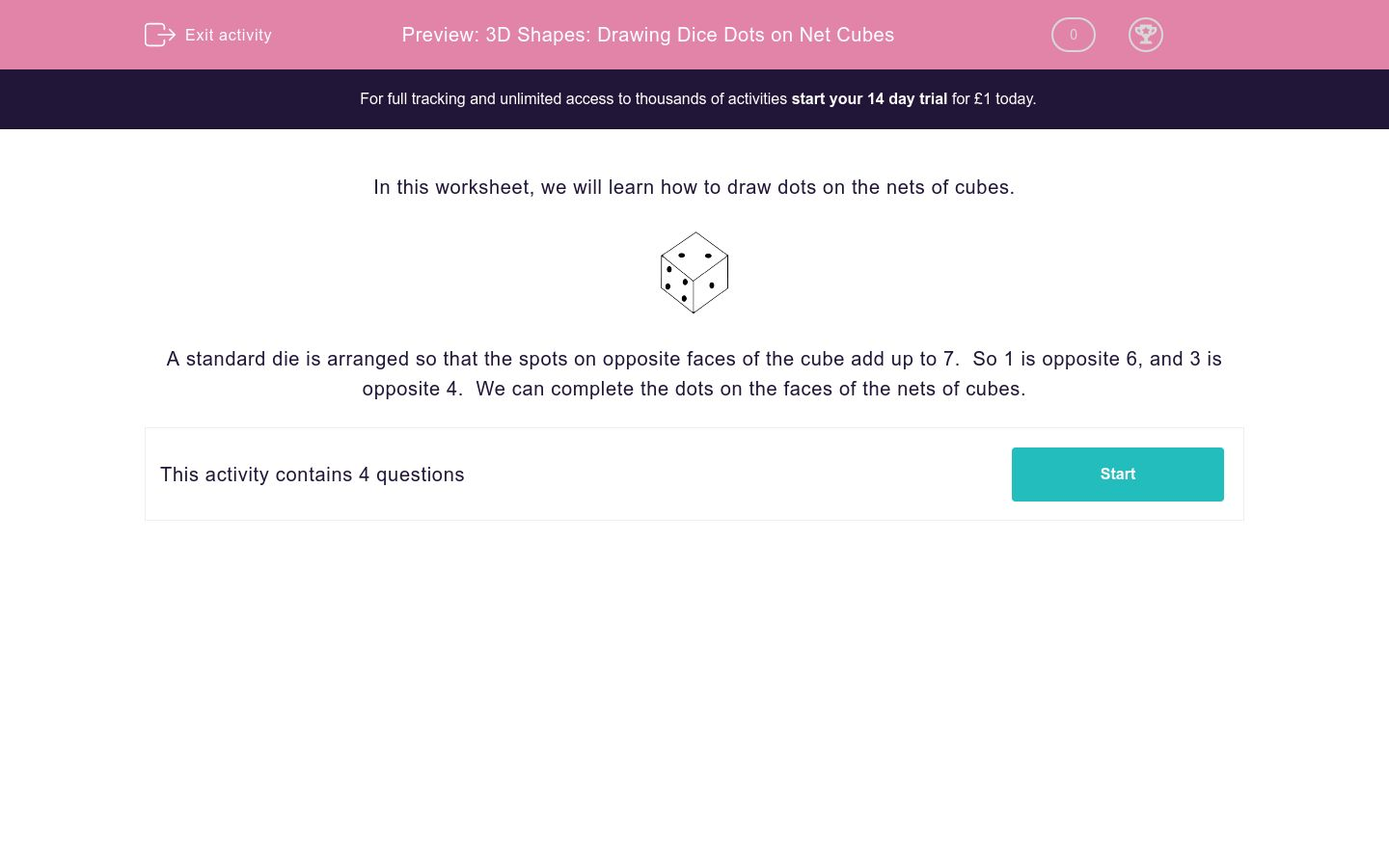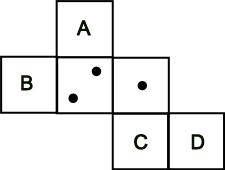# 3D Shapes: Drawing Dice Dots on Net Cubes

In this worksheet, students are directed to place dice dots and nets of cubes.Key stage:  KS 2

Curriculum topic:   Maths and Numerical Reasoning

Curriculum subtopic:   3D Shapes

Difficulty level:### QUESTION 1 of 10

In this worksheet, we will learn how to draw dots on the nets of cubes.A standard die is arranged so that the spots on opposite faces of the cube add up to 7.  So 1 is opposite 6, and 3 is opposite 4.  We can complete the dots on the faces of the nets of cubes.

If A = 1 and B = 2, work out what C and D must be.## Column B

C
5
D
6

If A = 3, work out what B, C and D must be.## Column B

B
5
C
6
D
4

If A =3 and B = 1, work out what C and D must be.## Column B

C
4
D
6

If A = 1 and B = 3, work out what C and D must be.## Column B

C
6
D
4
• Question 1

If A = 1 and B = 2, work out what C and D must be.## Column B

C
6
D
5
• Question 2

If A = 3, work out what B, C and D must be.## Column B

B
6
C
4
D
5
• Question 3

If A =3 and B = 1, work out what C and D must be.## Column B

C
4
D
6
• Question 4

If A = 1 and B = 3, work out what C and D must be.## Column B

C
6
D
4
---- OR ----